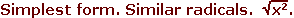Proyecto Salón Hogar

English SectionMath Page

# ALGEBRA

Home

Terms versus factors. The function of parentheses. The order of operations. The value of a letter. What it means to evaluate an expression.

The absolute value and the algebraic sign. Subtracting a larger number from a smaller. The number line. The negative of any number.

"Adding" a negative number. Naming terms. The rule for adding terms. Subtracting a negative number.

The Rule of Signs.

The rule of symmetry. Commutative rules. Inverses. Two rules for equations.

The Definition of Division. Rules for 0.

Parentheses. Brackets. Braces.
The relationship of  ab  to  ba.

Inverse operations. The four forms of equations. Transposing. A logical sequence of statements.

11.  Inequalities13.  Exponents I

Adding exponents. Power of a product. Multiplying exponents.

The definition of a polynomial in x.
Factoring polynomials.
Factoring by grouping. Equations that require factoring.

Products of binomials.

Quadratics in different arguments.

The square of a trinomial. Completing the square.

Summary of Multiplying/Factoring.
Factoring by grouping.
Factoring an ± bn.Rational expressions. The principle of equivalent fractions. Reducing to lowest terms.

Subtracting exponents. Negative exponents. Exponent 0. Scientific notation.

Complex fractions.

The Lowest Common Multiple (LCM) of a series of terms.

Clearing of fractions.

Same time problems: Upstream-downstream.
Job problems.Square roots. Equations x² = a. Rationalizing a denominator. Real numbers.Fractional radicand.

Conjugate pairs.

The index of a radical. Fractional exponent.

The real and imaginary components. Conjugate pairs.Actual versus potential infinities.

The distance of a point from the origin. The distance between any two points.

The equation of the first degree.

The point-slope formula. The two-point formula.

The method of addition. The method of substitution. Cramer's Rule. Three equations in three unknowns.

Investment problems. Mixture problems.
Upstream-downstream problems.

The roots of a quadratic. Solution by factoring. Completing the square. The quadratic formula.
The graph of a quadratic.38.  Logarithms

Definition. The three laws of logarithms. Common logarithms.39.  Variation

Direct variation. The constant of proportionality. Varies as the square. Varies inversely. Varies as the inverse square.

Home

www.proyectosalonhogar.com